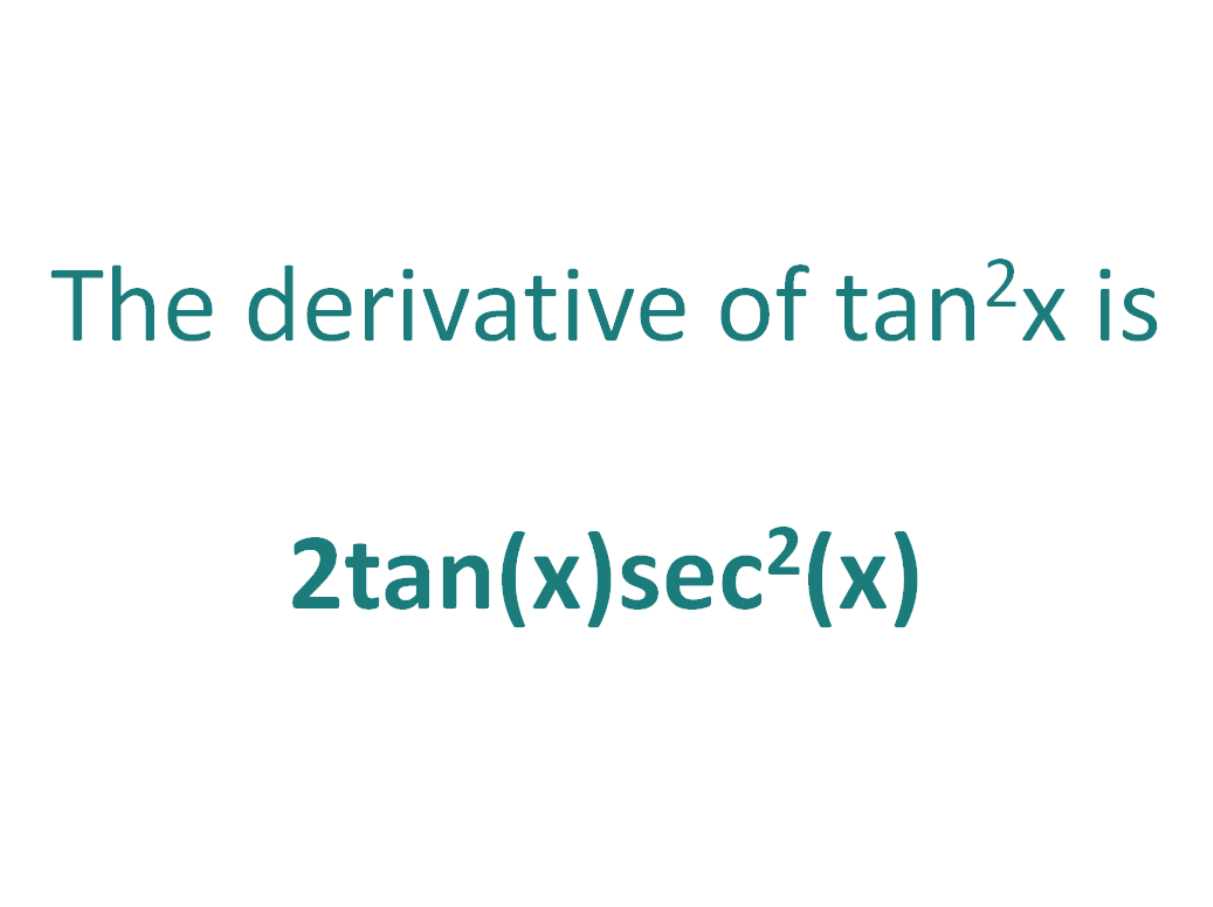# The Derivative of tan^2xThe derivative of tan^2x is 2.tan(x).sec^2(x)

## How to calculate the derivative of tan^2x

Note that in this post we will be looking at differentiating tan2(x) which is not the same as differentiating tan(2x). Here is our post dealing with how to differentiate tan(2x).

There are two methods that can be used for calculating the derivative of tan^2x.

The first method is by using the product rule for derivatives (since tan2(x) can be written as tan(x).tan(x)).

The second method is by using the chain rule for differentiation.

### Finding the derivative of tan^2x using the product rule

The product rule for differentiation states that the derivative of f(x).g(x) is f’(x)g(x) + f(x).g’(x)

The Product Rule:
For two differentiable functions f(x) and g(x)

If F(x) = f(x).g(x)

Then the derivative of F(x) is F'(x) = f’(x)g(x) + f(x)g'(x)

First, let F(x) = tan2(x)

Then remember that tan2(x) is equal to tan(x).tan(x)

So F(x) = tan(x)tan(x)

By setting f(x) and g(x) as tan(x) means that F(x) = f(x).g(x) and we can apply the product rule to find F'(x)

Using the product rule, the derivative of tan^2x is 2tan(x)sec2(x)

### Finding the derivative of tan^2x using the chain rule

The chain rule is useful for finding the derivative of a function which could have been differentiated had it been in x, but it is in the form of another expression which could also be differentiated if it stood on its own.

In this case:

• We know how to differentiate tan(x) (the answer is sec2(x))
• We know how to differentiate x2 (the answer is 2x)

This means the chain rule will allow us to perform the differentiation of the expression tan^2x.

#### Using the chain rule to find the derivative of tan^2x

Although the expression tan2x contains no parenthesis, we can still view it as a composite function (a function of a function).

We can write tan2x as (tan(x))2.

Now the function is in the form of x2, except it does not have x as the base, instead it has another function of x (tan(x)) as the base.

Let’s call the function of the base g(x), which means:

g(x) = tan(x)

From this it follows that:

tan(x)2 = g(x)2

So if the function f(x) = x2 and the function g(x) = tan(x), then the function (tan(x))2 can be written as a composite function.

f(x) = x2

f(g(x)) = g(x)2 (but g(x) = tan(x))

f(g(x)) = (tan(x))2

Let’s define this composite function as F(x):

F(x) = f(g(x)) = (tan(x))2

We can find the derivative of tan^2x (F'(x)) by making use of the chain rule.

The Chain Rule:
For two differentiable functions f(x) and g(x)

If F(x) = f(g(x))

Then the derivative of F(x) is F'(x) = f’(g(x)).g’(x)

Now we can just plug f(x) and g(x) into the chain rule.

How to find the derivative of tan^2x using the Chain Rule:

Using the chain rule, the derivative of tan^2x is 2.tan(x).sec2(x)

Finally, just a note on syntax and notation:tan^2x is sometimes written in the forms below (with the derivative as per the calculations above). Just be aware that not all of the forms below are mathematically correct.

## The Second Derivative Of tan^2x

To calculate the second derivative of a function, differentiate the first derivative.

From above, we found that the first derivative of tan^2x = 2tan(x)sec2(x). So to find the second derivative of tan^2x, we need to differentiate 2tan(x)sec2(x).

We can use the product and chain rules, and then simplify to find the derivative of 2tan(x)sec2(x) is 4sec2(x)tan2(x) + 2sec4(x)

The second derivative of tan^2x is 4sec2(x)tan2(x) + 2sec4(x)

## Interesting property of the derivative of tan^2x

It is interesting to note that the derivative of tan2x is equal to the derivative of sec2x.

The derivative of:
> tan2x = 2.sec2(x).tan(x)
> sec2x = 2.sec2(x).tan(x)